JEE  >  JEE Main Previous year questions (2016-22): Some Basic Concepts of Chemistry

# JEE Main Previous year questions (2016-22): Some Basic Concepts of Chemistry Notes | Study Chemistry for JEE - JEE

## Document Description: JEE Main Previous year questions (2016-22): Some Basic Concepts of Chemistry for JEE 2022 is part of Chemistry for JEE preparation. The notes and questions for JEE Main Previous year questions (2016-22): Some Basic Concepts of Chemistry have been prepared according to the JEE exam syllabus. Information about JEE Main Previous year questions (2016-22): Some Basic Concepts of Chemistry covers topics like and JEE Main Previous year questions (2016-22): Some Basic Concepts of Chemistry Example, for JEE 2022 Exam. Find important definitions, questions, notes, meanings, examples, exercises and tests below for JEE Main Previous year questions (2016-22): Some Basic Concepts of Chemistry.

Introduction of JEE Main Previous year questions (2016-22): Some Basic Concepts of Chemistry in English is available as part of our Chemistry for JEE for JEE & JEE Main Previous year questions (2016-22): Some Basic Concepts of Chemistry in Hindi for Chemistry for JEE course. Download more important topics related with notes, lectures and mock test series for JEE Exam by signing up for free. JEE: JEE Main Previous year questions (2016-22): Some Basic Concepts of Chemistry Notes | Study Chemistry for JEE - JEE
 1 Crore+ students have signed up on EduRev. Have you?

Q.1. To check the principle of multiple proportions, a series of pure binary compounds (PmQn)  were analyzed and their composition is tabulated below. The correct option(s) is(are)            (JEE Advanced 2022)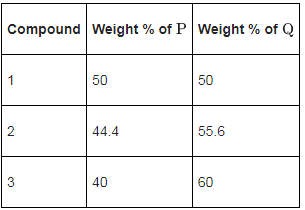(a) If empirical formula of compound 3 is P3Q4, then the empirical formula of compound 2 is P3Q5.
(b) If empirical formula of compound 3 is P3Q2 and atomic weight of element P is 20 , then the atomic weight of Q is 45.
(c) If empirical formula of compound 2 is PQ, then the empirical formula of the compound 1 is P5Q4.
(d) If atomic weight of P and Q are 70 and 35 , respectively, then the empirical formula of compound 1 is P2Q.

Ans. b, c

Q.2. The treatment of an aqueous solution of 3.74 g of Cu(NO3)2 with excess KI results in a brown solution along with the formation of a precipitate. Passing H2S through this brown solution gives another precipitate X. The amount of X (in g) is ___________.      (JEE Advanced 2022)
[Given: Atomic mass of H = 1, N = 14,O = 16, S = 32, K = 39, Cu = 63, I = 127]

Ans. 0.31 and 0.33

Q.3. Consider the reaction 4HNO3(1) + 3KCl(s) → Cl2(g) + NOCl(g) + 2H2O(g) + 3KNO3(s)
The amount of HNO3 required to produce 110.0 g of KNO3 is
(Given: Atomic masses of H,O,N and K are 1,16,14 and 39, respectively.)                      (2022)
(a) 32.2 g
(b) 69.4 g
(c) 91.5 g
(d) 162.5 g

Ans. c
4HNO3(1) + 3KCl(s) → Cl2(g) + NOCl(g) + 2H2O(g) + 3KNO3(s)
∵ 110 g of KNO3 ⇒ moles of KNO3 = 110/101
= 1.089 mol
As, 4 mole of HNO3 produces 3 mol of KNO3.
Hence, the moles of HNO3 required to produce
1.089 moles of KNO3 = 4/3 ×1.089=1.452 mol
Hence, mass of HNO3 required is 1.452 × 63
= 91.5 g

Q.4.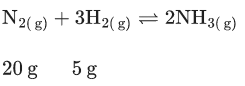Consider the above reaction, the limiting reagent of the reaction and number of moles of NH3 formed respectively are :                      (2022)

(a) H2,1.42 moles
(b) H2,0.71 moles
(c) N2,1.42 moles
(d) N2,0.71 moles

Ans. c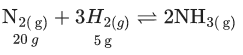Ideally 28 g N2 reacts with 6 gH2 limiting reagent is N2
∴ Amount of NH3 formed on reacting 20 g N2 is, moles 20 g N2 is,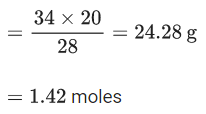Q.5. In Carius method of estimation of halogen, 0.45 g of an organic compound gave 0.36 g of AgBr. Find out the percentage of bromine in the compound.
(Molar masses : AgBr = 188 g mol−1; Br = 80 g mol−1)                      (2022)
(a) 34.04%
(b) 40.04%
(c) 36.03%
(d) 38.04%

Ans. a
Mass of organic compound = 0.45gm
Mass of AgBr obtained = 0.36gm
∴ Moles of AgBr = 0.36/188
∴ Mass of Bromine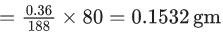∴ % Br in compound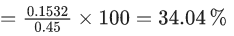Q.6. 250 g solution of D-glucose in water contains 10.8% of carbon by weight. The molality of the solution is nearest to
(Given: Atomic Weights are, H, 1u;C, 12u; O, 16u)                      (2022)
(a) 1.03
(b) 2.06
(c) 3.09
(d) 5.40

Ans. b
Weight of D-glucose in water
= 250 g
Weight of carbon in D-glucose
∴ Weight of carbon in D-glucose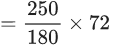= 100 g
% of carbon in the aqueous solution of glucose is =10.8%
∴ Weight of the solution is = 925.93
Molality of D-glucose is
∴ Molality of D-glucose is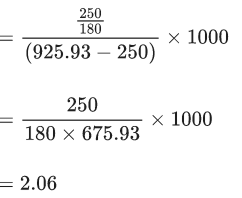Q.7. Hemoglobin contains 0.34% of iron by mass. The number of Fe atoms in 3.3 g of hemoglobin is
(Given: Atomic mass of Fe is 56u, NA = 6.022 × 1023 mol−1.)                      (2022)
(a) 1.21 × 105
(b) 12.0 × 1016
(c) 1.21 × 1020
(d) 3.4 × 1022

Ans. c
According to the question,
100 g of hemoglobin contains 0.34 g of iron
3.3 g of hemoglobin contains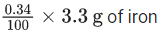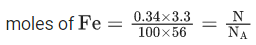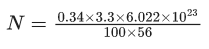= 1.21 x 1020

Q.8. SO2Cl2 on reaction with excess of water results into acidic mixture SO2Cl2 + 2H2O → H2SO4 + 2HCl
16 moles of NaOH is required for the complete neutralisation of the resultant acidic mixture. The number of moles of SO2Cl2 used is :                     (2022)
(a) 16
(b) 8
(c) 4
(d) 2

Ans. c
SO2Cl2 + 2H2O → H2SO+ 2HCl
Moles of NaOH required for complete neutralization of resultant acidic mixture =16 moles
And 1 mole of SO2Cl2 produced 4 moles of H+.
∴ Moles of SO2Cl2 used will be = 16/4 = 4 moles

Q.9. If a rocket runs on a fuel (C15H30) and liquid oxygen, the weight of oxygen required and CO2 released for every litre of fuel respectively are :
(Given : density of the fuel is 0.756 g/mL)                      (2022)

(a) 1188 g and 1296 g
(b) 2376 g and 2592 g
(c) 2592 g and 2376 g
(d) 3429 g and 3142 g

Ans. c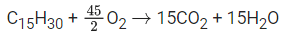Given, volume of fuel = 1L = 1000 ml
And density of fuel = 0.756 g/ml
We know,
d = w/v
⇒ 0.756 = w/1000
⇒ w = 756 gm
∴ weight of fuel = 756 gm
Molar mass of C15H30 = 15 × 12 + 30 = 210
∴ Moles of C15H30 = 756/210
From equation you can see,
1 mole of C15H30 react with 45/2 mole of O2
∴ 756/210 moles of C15H30 react with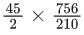moles of O2
∴ Moles of O2 required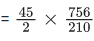∴ Mass of O2 required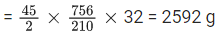Also,
From 1 mole of C15H30 15 moles of CO2 formed
∴ From 756/210 moles of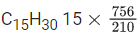moles of CO2 formed
∴ Moles of CO2 formed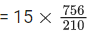∴ Mass of CO2 formed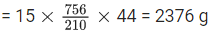Q.10. 120 g of an organic compound that contains only carbon and hydrogen gives 330 g of CO2 and 270 g of water on complete combustion. The percentage of carbon and hydrogen, respectively are                      (2022)
(a) 25 and 75
(b) 40 and 60
(c) 60 and 40
(d) 75 and 25

Ans. d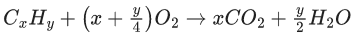From the reaction,
Produced CO2 = x mol
and produced H2O = y2 mol
Given produced CO2 = 330 g
∴ moles of CO2 = 330/44 = 30/4 = x
Also given produced H2O = 270 gm
∴ Moles of H2O = 270/18 = 15 = y/2.
⇒ y = 30
∴ x : y = 30/4 :30 = 1:4
Formula of the compound = (CH4)n
∴ Weight of C in (CH4)n = 12 n
Weight of H in (CH4)n = 4 n
∴ Weight ratio of C and H
= 12 n : 4 n
= 3 : 1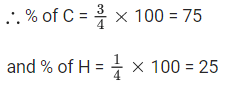Q.11. A commercially sold conc. HCl is 35% HCl by mass. If the density of this commercial acid is 1.46 g/mL, the molarity of this solution is:
(Atomic mass : Cl = 35.5 amu, H = 1 amu)                       (2022)
(a) 10.2 M
(b) 12.5 M
(c) 14.0 M
(d) 18.2 M

Ans. c
35% HCl by mass means in 100 gm HCl solution 35 gm HCl present.
Now, volume of 100 gm HCl solution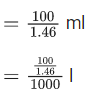Moles of HCl = 35/36.5
Now, molarity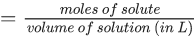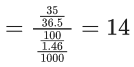Q.12.  Compound A contains 8.7% Hydrogen, 74% Carbon and 17.3% Nitrogen. The molecular formula of the compound is,
Given : Atomic masses of C, H and N are 12, 1 and 14 amu respectively.
The molar mass of the compound A is 162 g mol−1.                      (2022)
(a) C4H6N2
(b) C2H3N
(c) C5H7N
(d) C10H14N2

Ans. d
Mole ratio of H, C and N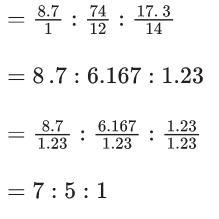∴ Emperical formula = C5H7N
∴ Molecular formula =(C5H7N)n
Given molecular mass = 162
Molecular mass of (C5H7N)n
=(5 × 12 + 7 × 1 + 14) × n
= (81) × n
∴ 81 × n = 162
⇒ n = 2
∴ Molecular formula = C10H14N2

Q.13. Using the rules for significant figures, the correct answer for the expression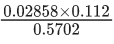will be                      (2022)
(a) 0.005613
(b) 0.00561
(c) 0.0056
(d) 0.006

Ans. b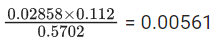Reported answer should not be more precise than least precise term (0.112 is the least precise term with three significant figures) in calculations, so there should be three significant figures in reported answer.

Q.14. Production of iron in blast furnace follows the following equation
Fe3O4(s) + 4CO(g) → 3Fe(l) + 4CO2(g)
when 4.640 kg of Fe3O4 and 2.520 kg of CO are allowed to react then the amount of iron (in g) produced is :
[Given : Molar Atomic mass (g mol−1) : Fe = 56, Molar Atomic mass (g mol−1) : O = 16, Molar Atomic mass (g mol−1) : C = 12]                       (2022)
(a) 1400
(b) 2200
(c) 3360
(d) 4200

Ans. c
Given,
Mass of Fe3O4 = 4.640 kg = 4640 gm
Molar mass of Fe3O4 = 56 × 3 + 16 × 4 = 232 g
∴ Moles of Fe3O= 4640/232 = 20
Also, given
Mass of CO = 2.520 kg = 2520 gm
Molar mass of CO = 12 + 16 = 28 gm
∴ Molar of CO = 2520/28 = 90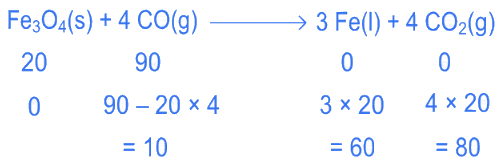Here Fe3O4 is limiting reagent as to find limiting reagent, divide the given moles of reactants with their respective stoichiometric coefficient and reactant for which this ratio is minimum will be limiting reagent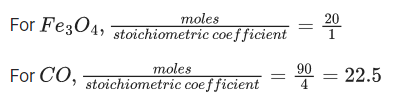∴ Fe3O4 is limiting reagent.
Now produced Fe = 20 × 3 = 60 mol
∴ Weight of Fe = 60 × 56 = 3360 g

Q.15. A 1.84 mg sample of polyhydric alcoholic compound 'X' of molar mass 92.0 g/mol gave 1.344 mL of H2 gas at STP. The number of alcoholic hydrogens present in compound 'X' is ________.                     (2022)

Ans. 3
Volume of H2 gas =1.344 mL.
Mole of H2 gas =1.344 / 22400 = 6 × 10−5.
No of H atoms per molecule of H= 2.
No. of moles of organic compound =1.84×10−3 / 92 = 2×10−5
No. of −OH (hydroxyl group in one molecule)   = 6 × 10-5 / 2 × 10-5 = 3

Q.16. A 100 mL solution of CH3CH2MgBr on treatment with methanol produces 2.24 mL of a gas at STP. The weight of gas produced is _____________ mg. [nearest integer] (Round off to the Nearest Integer).            (2022)

Ans.  3
CH3−CH2−MgBr+CH3OH → CH3−CH3+ MgBr(OCH3)
As 2.24 ml is formed at STP.
Number of moles (M) of ethane gas produced:
= 2.24 x 10-4 / 22.4
= 10−4 ml
Weight of ethane produced = n x M =10-4 × 30 = 3 mg.

Q.17. 20 mL of 0.02M hypo solution is used for the titration of 10 mL of copper sulphate solution, in the presence of excess of KI using starch as an indicator. The molarity of Cu2+ is found to be ____________ ×10−2M. [nearest integer]            (2022)

Ans. 4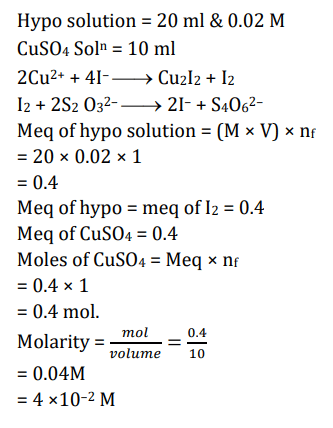Q.18. When 800 mL of 0.5 M nitric acid is heated in a beaker, its volume is reduced to half and 11.5 g of nitric acid is evaporated. The molarity of the remaining nitric acid solution is x × 10−2 M. (Nearest integer) (Molar mass of nitric acid is 63 g mol−1)       (2022)

Ans.  54
m moles of HNO= 800 × 0.5
Moles of HNO= 400×10−3= 0.4 moles
Weight of HNO= 0.4×63 g = 25.2 g
Remaining acid = 25.2−11.5 = 13.7 g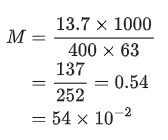Q.19. Chlorophyll extracted from the crushed green leaves was dissolved in water to make 2 L solution of Mg of concentration 48ppm. The number of atoms of Mg in this solution is x×1020 atoms. The value of x is ___________. (Nearest Integer)(Given : Atomic mass of Mg is 24 g mol−1;NA=6.02×1023 mol−1 )       (2022)

Ans. 24
In 2L→96mg of Mg
Number of atoms of Mg = 96 × 10−3 / 24 × NA
=4×10−3×6×1023 = 24×1020
x = 24

Q.20. A sample of 4.5 mg of an unknown monohydric alcohol, R-OH was added to methylmagnesium iodide. A gas is evolved and is collected and its volume measured to be 3.1 mL. The molecular weight of the unknown alcohol is __________ g/mol. [Nearest integer]  (2022)

Ans. 33
R−OH+CH3Mgl ⇒ R−OMgl+CH4
moles of alcohol (ROH) ≡  moles of CH4
At STP,      [Assuming STP]
1 mole corresponds to 22.7 L
Hence, 3.1 mL ≡ 3.1 / 22700 mol
So, moles of alcohol = 3.1 / 22700
⇒ 3.1 / 22700 = 4.5×10−3 / M
M = 33 g/mol

Q.21. 56.0 L of nitrogen gas is mixed with excess hydrogen gas and it is found that 20 L of ammonia gas is produced. The volume of unused nitrogen gas is found to be _________ L.        (2022)

Ans. 46
N2( g)+3H2( g)→2NH3( g)
Since H2 is in excess and 20 L of ammonia gas is produced.
Hence, 2 moles NH≡ 1 mole N(v∝n)
20 LNH≡ 10 L N2
Volume of N2 left = 56−10 = 46 L.

Q.22. Number of grams of bromine that will completely react with 5.0 g of pent-1-ene is ___________ × 10−2 g. (Atomic mass of Br = 80 g/mol) [Nearest Integer]                 (2022)

Ans. 1143
Molar mass of C5H10 = 12 × 5 + 10 = 70 gm
Given mass of C5H10 = 5 gm
∴ Moles of C5H10 = 570
From reaction,
1 mole of C5H10 reacts with 1 mole of Br2
∴ 5/70 moles of C5H10 reacts with 5/70 moles of Br2
∴ Reacted Br2 = 5/70 × 160 gm
= 11.428 gm
= 1142.8 × 10−2 gm
≃ 1143 × 10−2 gm

Q.23. The number of N atoms in 681 g of C7H5N3O6 is x × 1021. The value of x is (NA = 6.02 × 1023 mol−1) (Nearest Integer)                   (2022)

Ans. 5418
Molar mass of C7H5N3O6
= 12 × 7 + 5 + 14 × 3 + 16 × 6
= 227 gm
Given mass of C7H5N3O6 = 681 gm
∴ Moles of C7H5N3O6 = 681 / 227=3
In one molecule of C7H5N3O6, 3 N atoms present.
∴ In 1 mole C7H5N3O6, 3 moles of N atoms presents.
∴ In 3 moles of C7H5N3O6, 3 × 3 = 9 moles of N atoms presents.
We know, 1 mole of N atoms = 6.02 × 1023 N atoms.
∴ 9 moles of N atoms = 9 × 6.02 × 1023 = 54.18 × 1023 = 5418 × 1021

Q.24. The complete combustion of 0.492 g of an organic compound containing 'C', 'H' and 'O' gives 0.793 g of CO2 and 0.442 g of H2O. The percentage of oxygen composition in the organic compound is ______________. (nearest integer)                      (2022)

Ans. 46
Total organic compound = 0.492 gm
Produced CO2 = 0.793 gm
∴ Moles of CO2 = 0.793/44
∴ Moles of C atoms = 0.793/44
∴ Weight of C atoms = 0.79344 × 12 = 0.216 g
Produced H2O = 0.442 gm
∴ Moles of H2O = 0.442/18
∴ Moles of H atoms = 0.442/18
∴ Weight of H atoms = 0.442/18 × 2 = 0.05 g
∴ Weight of O atoms
= 0.492 − (0.216 + 0.05)
= 0.226 gm
% by mass of oxygen in compound
= 0.226/0.492 × 100 = 46%

Q.25. Complete combustion of of an organic compound provides of and of The percentage composition of carbon and hydrogen in organic compound is 15.3 and ______ respectively. (Round off to the Nearest Integer)             (2021)

Ans. 3%
44 gm CO2 have 12 gm carbon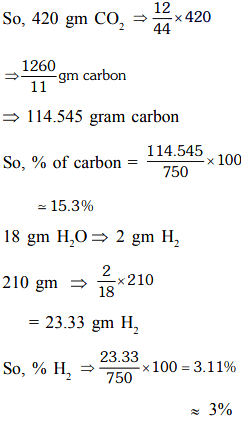Q.26. A 6.50 molal solution of KOH (aq.) has a density of . The molarity of the solution is_____ mol dm-3 (Round off to the Nearest Integer).              (2021)

Ans. 9.00
By using the relation,
m = 1000 × M / (1000 × d) − M × Msolute
Where, m = molality of the solution = 6.50 m
M = molarity of the solution
d = density = 1.89 g cm−3
Msolute = Molar mass of solute = 39+16+1 = 56 u
Substituting the values, we get
6.5 = 1000 × M / 1890 −M × 56
⇒ 12285−364M = 1000M
⇒ 1364M = 12285
⇒ M = 9 mol dm−3

Q.27. The mole fraction of a solute in a 100 molal aqueous solution ____  x 10-2 (Round off to the Nearest Integer).                           (2021)

Ans. 64
Let weight of H2O = 1000 g
Moles of solute = 100
(mole)H2O = 1000/18
Mole fraction of solute = mole of solute / Total moles = 1800 / 2800
Xsolute = 64 × 10-2

Q.28. Complete combustion of 3g of ethane gives x × 1022 molecules of water. The value of x is __________. (Round off to the Nearest Integer). [Use : NA = 6.023 × 1023; Atomic masses in u : C : 12.0; O : 16.0; H : 1.0]                       (2021)

Ans. 18
C2H6 + O2 → 2CO2 + 3H2O
3gm 0.3 mol
0.1 mol 0.3 NA
= 0.3 × 6.023 × 1023 molecules of H2O
= 1.8069 × 1023
= 18.069 × 1022

Q.29. A reaction of 0.1 mole of Benzylamine with bromomethane gave 23 g of Benzyl trimethyl ammonium bromide. The number of moles of bromomethane consumed in this reaction are n × 10−1, when n = __________. (Round off to the Nearest Integer). (2021)

Ans. 3
Number of moles of benzyl trimethyl
ammonium bromide formed = 23 / 230 = 0.1
∴ No. of moles of bromomethane consumed
= 3 × 0.1
= 3 × 10–1

Q.30. Consider the reaction below. The percentage yield of amide product is __________. (Round off to the Nearest Integer). (Given : Atomic mass : C : 12.0 u, H : 1.0 u, N : 14.0 u, O : 16.0 u, Cl : 35.5 u)                        (2021)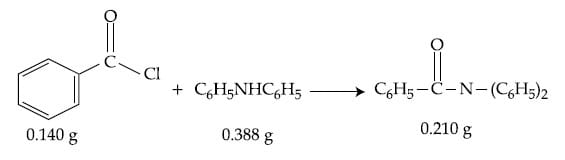Ans. 77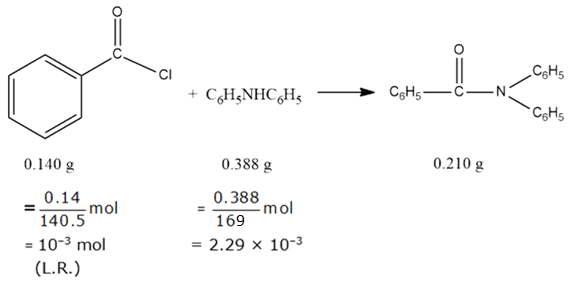Stoichiometric moles of amide = 10–3 mol
Actual moles of amide = 7.69 × 10–4 mol
% yield = 7.69×10-4×100 / 10-3
= 76.9%
≃ 77%

Q.31. If the concentration of glucose (C6H12O6) in blood is 0.72 g L−1, the molarity of glucose in blood is ____________ × 10−3 M. (Nearest integer)  [Given : Atomic mass of C = 12, H = 1, O = 16 u]         (2021)

Ans. 4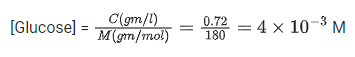Q.32. When 0.15 g of an organic compound was analyzed using Carius method for estimation of bromine, 0.2397 g of AgBr was obtained. The percentage of bromine in the organic compound is ______________. (Nearest integer)   [Atomic mass : Silver = 108, Bromine = 80]      (2021)

Ans.  68
Moles of Br = Moles of AgBr obtained
⇒ Mass of Br = 0.2397 / 188 × 80 g
Therefore, percentage Br in the organic compound
= WBr / WT × 100
0.2397 × 80 / 188 × 0.15 × 100 = 0.85 × 80 = 68
⇒ Nearest integer is '68'.

Q.33. 100 g of propane is completely reacted with 1000 g of oxygen. The mole fraction of carbon dioxide in the resulting mixture is x × 10−2The value of x is ____________. (Nearest integer) [Atomic weight : H = 1.008; C = 12.00; O = 16.00]          (2021)

Ans. 19
100 g of propane = 2.27mol
1000 g oxygen = 31.25 mol
C3H8(g)+5O2(g)→3CO2(g)+4H2O(l)
From the equation 1 mol of propane reacts with 5 mol of oxygen to give 3 moles of carbon dioxide and 4 moles of water
So, by stoichiometric calculations:
2.27 mol of propane will react with 11.35 mol oxygen to give 6.81 mol carbon dioxide and 9.08 mol water
C3H8(g)+5O2(g)→3CO2(g)+4H2O(l)
t=0     2.27           31.25            0                  0
t=∞       0                19.9           6.81           9.08
mole fraction of CO2 in the final reaction mixture (heterogenous)
XCO= 6.81 / 19.9+6.81+9.08
= 0.1902 =19.02 × 10−2
Value of x is 19

Q.34. An average person needs about 10000 kJ energy per day. The amount of glucose (molar mass = 180.0 g mol−1) needed to meet this energy requirement is ____________ g.
(Use : ΔCH(glucose) = 2700 kJ mol−1)                       (2021)

Ans. 667
1 mole glucose give 2700 kJ energy,
so mole of glucose needed for 104 kJ energy
= 10000 / 2700 = 3.703 moles
Weight of glucose = 3.703 × 180 g/moles
= 666.666
≈ 667 g
Hence, amount of glucose required is 667 g.

Q.35. The ammonia (NH3) released on quantitative reaction of 0.6 g urea (NH2CONH2) with sodium hydroxide (NaOH) can be neutralized by    (2020)
(a) 200 mL of 0.4 N HCl
(b) 200 mL of 0.2 N HCl
(c) 100 mL of 0.2 N HCl
(d) 100 mL of 0.1 N HCl

Ans. c
From the stoichiometry of the reaction,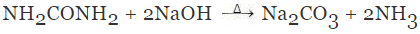1 mol of urea liberates 2 moles of ammonia
Moles of urea = 0.6/60 = 0.01 mol
Thus, moles of ammonia formed = 2 × 0.01 = 0.02 mol
Since, 1 mol HCl is required to neutralized 1 mol of ammonia. So, 0.02 moles of HCl is required to neutralized 0.02 moles of ammonia.
From the given concentration of HCl, 100 mL of 0.2 N is equivalent to 0.02 moles of ammonia.
⇒ Moles of HCl = 0.1 × 0.2 = 0.02 moles

Q.36. Ferrous sulphate heptahydrate is used to fortify foods with iron. The amount (in grams) of the salt required to achieve 10 ppm of iron in 100 kg of wheat is ______.    (2020)
Atomic weight: Fe = 55.85; S = 32.00; O = 16.00

Ans. 4.95
The mass of the ferrous sulphate heptahydrate (FeSO. 7H2O) = 277.55 g mol-1
⇒ 277.55 g of (FeSO4 . 7H2O) 55.85 g of Fe is present.
⇒ 1 g of Fe is present in = 277.55/55.85 g of FeSO4 . 7H2O
⇒1 g of Fe is present in = 4.97 g of FeSO4.7H2O

Q.37. The molarity of HNO3 in a sample which has density 1.4 g mL-1 and mass percentage of 64% is ______. (Molecular weight of HNO3 = 63).         (2020)

Ans. 14
Given,
ρ =1.4 g mL-1 and mass percentage = 63%
We know,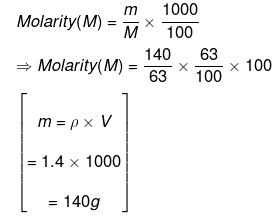⇒ Molarity (M) = 14 mol L-1

Q.38. 10.30 mg of O2 is dissolved into a liter of sea water of density 1.03 g mL-1. The concentration of O2 in ppm is ______.         (2020)

Ans. 10.0
The concentration of dissolved oxygen in water is
DO in water (ppm)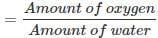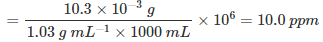Q.39. Amongst the following statements, that which was not proposed by Dalton was        (2020)
(a) chemical reactions involve reorganization of atoms. These are neither created nor destroyed in a chemical reaction.
(b) all the atoms of a given element have identical properties including identical mass. Atoms of different elements differ in mass.
(c) when gases combine or reproduced in a chemical reaction, they do so in a simple ratio by volume provided all gases are at the same T and P.
(d) matter consists of indivisible atoms.

Ans. c
The statement (c) is incorrect and the correct statement is:
When atoms of different elements combine to form compounds, new and more complex particles form.
However, in a given compound the constituent atoms are always present in the same fixed numerical ratio.

Q.40. The strength of an aqueous NaOH solution is most accurately determined by titrating (Note: Consider that an appropriate indicator is used)    (2020)
(a) Aq. NaOH in a pipette and aqueous oxalic acid in a burette.
(b) Aq. NaOH in a burette and aqueous oxalic acid in a conical flask.
(c) Aq. NaOH in a burette and concentrated H2SO4 in a conical flask.
(d) Aq. NaOH in a volumetric flask and concentrated H2SO4 in conical flask.

Ans. b
The strength of aq. NaOH solution is most accurately determined by titration with standard oxalic acid. Keeping aq. NaOH in burette and oxalic acid in conical flask.

Q.41. NaClO3 is used, even in spacecrafts, to produce O2. The daily consumption of pure O2 by a person is 492 L at 1 atm, 300 K. How much amount of NaClO3, in grams, is required to produce O2 for the daily consumption of a person at 1 atm, 300 K?    (2020)
NaClO3(s) + Fe(s) → O2(g) + NaCl(s) + FeO(s)
R = 0.082 L atm mol−1 K−1

Ans. 2130
The given reaction is
NaClO3(s) + Fe(s) → FeO(s) + NaCl(s) + O2(g)
From the above reaction,
1 mol of oxygen is produced by 1 mol of NaClO3,
16 g of oxygen is produced by 106.5 g of NaClO3,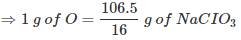Amount of O2 required by a person in a day in grams can be calculated by ideal gas equation,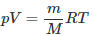⇒ m = pVM/RT
Substituting the values in Eq. (1), we get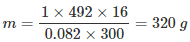Thus, 320 g of oxygen is produced by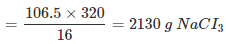Q.42. The mass percentage of nitrogen is histamine is ______.      (2020)

Ans. (37.80)
The structural formula of histamine is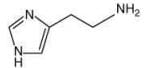The molar mass of histamine (C5H9N3) = 111.15 g mol–1
Thus, the mass percentage of nitrogen in histamine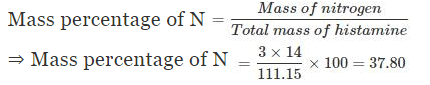Q.43. The hardness of a water sample containing 10−3 M MgSO4 expressed as CaCO3 equivalents (in ppm) is ______.    (2020)
(Molar mass of MgSO4 is 120.37 g mol–1)

Ans. (100)
We know, 10–3 M of MgSO4 = 0.001 moles of MgSO4 present in 1 L water.
Since, number of moles of MgSO4 and CaCO3 are equal, that is, nMgSO4 = nCaCO3.
Thus, hardness of water sample in expressed as CaCO3 equivalents in ppm is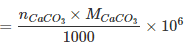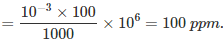Q.44. 5 g of zinc is treated separately with an excess of
(i) dilute hydrochloric acid and

(ii) aqueous sodium hydroxide
The ratio of the volumes of H2 evolved in these two reactions is        (2020)
(a) 1 : 2
(b) 1 : 1
(c) 1 : 4
(d) 2 : 1

Ans. b
The moles of zinc nzs = m/M = 5/65.38 = 0.076 mol
The reaction of zinc with HCI and NaOH is
Zn(s) + 2HCI(aq) → ZnCI2(aq)+H2(g)
Zn(s) + 2NaOH(aq) + 2H2O(I) → Na2Zn(OH)4(aq) + H2(g)
1 mol of Zn react with HCI and NaOH respectively, 1 mol of hydrogen gas is liberated from each reaction. Thus, the ratio of hydrogen gas evolved when 5 g of Zn reacts with dil. HCI and aq. NaOH solutions is 1:1.

Q.45. The redox reaction among the following is    (2020)
(a) Formation of ozone from atmospheric oxygen in the presence of sunlight
(b) Reaction of [Co(H2O)6]Cl3 with AgNO3
(c) Reaction of H2SO4 with NaOH
(d) Combination of dinitrogen with dioxygen at 2000 K

Ans. d
The reaction in which both oxidation and reduction takes place are called redox reaction. Amongst the given, option (4) is a example of redox reaction.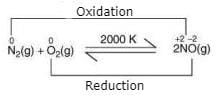Q.46. A solution of sodium sulphate contains 92 g of Na+ ions per kilogram of water. The molality of Na+ ions in that solution in mol kg-1 is:    (2019)
(a) 12
(b) 4
(c) 8
(d) 16

Ans. b
Number of moles in 92 g of Na+ = 92/23 = 4 moles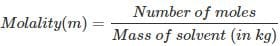∴ m = 4/1 = 4 mol kg-1

Q.47. For the following reaction the mass of water produced from 445 g of C57H110O6 is:      (2019)
2C57H110O6(s) + 163 O2(g) → 114CO2(g) + 110 H2O(1)
(a) 490 g
(b) 445 g
(c) 495 g
(d) 890 g

Ans. c
For the given reaction:
2C57H110O6(s) + 163 O2(g) →114 CO2(g) + 110 H2O(1)
n = 445/890 = 0.5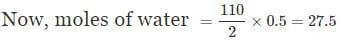∴ Mass of water = 27.5 × 18 = 495 g

Q.48. A mixture of 100 milli mol of Ca(OH)2 and 2 g of sodium sulphate was dissolved in water and the volume was made up to 100 mL. The mass of calcium sulphate formed and the concentration of OH- in resulting solution, respectively, are: (Molar mass of Ca(OH)2, Na2SO4 and CaSO4 are 74, 143 and 136 g mol-1, respectively; Ksp of Ca(OH)2 is 5.5 x 10-6)    (2019)
(a) 1.9 g, 0.28 mol L-1
(b) 13.6 g, 0.28 mol L-1
(c) 1.9 g, 0.14 mol L-1
(d) 13.6 g, 0.14 mol L-1

Ans. a
Na2SO4 + Ca(OH)→ CaSO4 + 2NaOH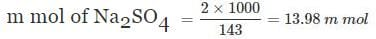m mol of CaSOformed = 13.98 m mol
Mass of CaSO4 formed
= 13.98 × 10-3 × 136 = 1.90 g
m mol of NaOH = 28 m mol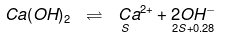Value of 'S' will be negligible so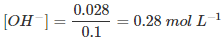Q.49. A 10 mg effervescent tablet containing sodium bicarbonate and oxalic acid releases 0.25 mL of CO2 at T = 298.15K and P = 1 bar. If molar volume of CO2 is 25.0 L under such condition, what is the percentage of sodium bicarbonate in each tablet?    (2019)
[Molar mass of NaHCO3 = 84 g mol-1]
(a) 0.84
(b) 33.6
(c) 16.8
(d) 8.4

Ans. d
2NaHCO3 + H2C2O4 → Na2C2O4 + 2CO2 + 2H2O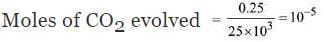∴ Moles of NaHCO3 = 10-5
∴ Mass of NaHCO3 = 84 × 10-5 g
= 0.84 × 10-3 g
= 0.84 mg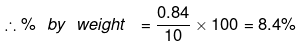Q.50. 25 mL of the given HCI solution requires 30 mL of 0.1 M sodium carbonate solution. What is the volume of this HCI solution required to titrate 30 mL of 0.2 M aqueous NaOH solution?    (2019)
(a) 25 mL
(b) 75 mL
(c) 50 mL
(d) 12.5 mL

Ans. a
Eq. of HCL = Eq. of Na2CO3
25 × N = 2 × 0.1 × 30
HCl = 0.24 N
Eq. of HCl = eq. of NaOH
0.24 × V = 30 × 0.2
V = 25 ml

Q.51. 50mL of 0.5 M oxalic acid is needed to neutralize 25 mL of sodium hydroxide solution. The amount of NaOH in 50 mL of the given sodium hydroxide solution is:    (2019)
(a) 4 g
(b) 1 g
(c) 2 g
(d) 8 g

Ans. Bonus (No option is correct)
Mili equivalents of oxalic acid = mili equivalent of sodium hydroxide
⇒ 50 × 0.5 × 2 = 25 × M × 1
⇒ M = 2 molar
Mass of NaOH in 50ml solution
= 50/1000 × 2 × 40 = 4g

Q.52. For a reaction, N2(g) + 3H2(g) → 2 NH3(g); identify dihydrogen (H2) as a limiting reagent in the following reaction mixtures.    (2019)
(a) 56 g of N2 + 10 g of H2
(b) 35 g of N2 + 8 g of H2
(c) 28 g of N2 + 6 g of H2
(d) 14 g of N2 + 4 g of H2

Ans. a
According to the stoichiometry of balanced equation 28 g N2, react with 6 g H2,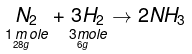∴ For 56 g of N2, 12 g of H2 is required

Q.53. At 300 K and 1 atmospheric pressure, 10 mL of a hydrocarbon required 55 mL of O2 for complete combustion, and 40 mL of CO2 is formed. The formula of the hydrocarbon is:    (2019)
(a) C4H10
(b) C4H6
(c) C4H7C1
(d) C4H8

Ans. b
Let the hydrocarbon be CxHy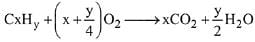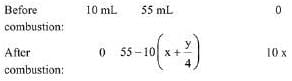Volume of CO2, 10x = 40; x = 4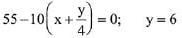∴ Hydrocarbon is C4H6

Q.54. The minimum amount of O2(g) consumed per gram of reactant is for the reaction: (Given atomic mass: Fe = 56, O= 16, Mg = 24, P = 31, C = 12, H = 1)         (2019)
(a) 4 Fe(s) + 3O2(g) → 2 Fe2O3 (s)
(b) P4(s) + 5O2(g) → P4O10(s)
(c) C3H8(g) + 5O2(g) → 3CO2(g) + 4H2O(I)
(d) 2Mg(s) + O2(g) → 2MgO(s)

Ans. a
(1) 4Fe + 3O2 → 2Fe2O3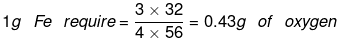(2) P4 + 5O2 → P4O10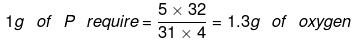(3) C3H8 + 5O2 → 3CO2 + 4H2O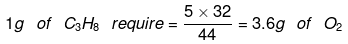(4) 2Mg + O2 → 2MgO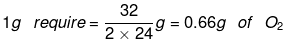Q.55. 5 moles of ABweigh 125 x 10-3 kg and 10 moles of A2B2 weigh 300 x 10-3 kg. The molar mass of A (MA) and molar mass of B (MB) in kg mol-1 are:    (2019)
(a) M= 10
× 10-3 and MB = 5 × 10-3
(b) M= 50
× 10-3 and M= 25 × 10-3
(c) MA = 25
× 10-3 and M= 50 × 10-3
(d) MA = 5
× 10-3 and MB = 10 × 10-3

Ans. d
5 mol AB, weighs 125 g
∴ AB2 = 25 g/mol
10 mol A2B2 weighs 300 g
∴ A2B2 = 30 g/mol
∴ Molar mass of A(MA) = 5 g or 5 × 10-3 kg
Molar mass of B(MB) = 10 g or 10 × 10-3 kg

Q.56. Consider the following reduction processes:
Zn2+ + 2e- → Zn(s); E° = - 0.76 V
Ca2+ + 2e- → Ca(s); E° = - 2.87 V
Mg2+ + 2e- → Mg(s); E° = - 2.36 V
Ni2+ + 2e- → Ni(s); E° = - 0.25 V
The reducing power of the metals increases in the order:    (2019)
(a) Ca < Zn < Mg < Ni
(b) Ni < Zn < Mg < Ca
(c) Zn < Mg < Ni < Ca
(d) Ca < Mg < Zn < Ni

Ans. b
Higher the oxidation potential, higher will be the reducing power. So, the order of reducing behaviour is:
Ca > Mg > Zn > Ni

Q.57. In the reaction of oxalate with permanganate in acidic medium, the number of electrons involved in producing one molecule of CO2 is:    (2019)
(a) 1
(b) 10
(c) 2
(d) 5

Ans. (a)
Reaction involved: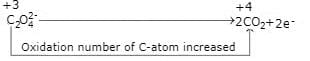∴ The number of electrons involved in producing one mole of CO2 is 1.

Q.58. In order to oxidise a mixture of one mole of each of FeC2O4, Fe2(C2O4)3, FeSO4 and Fe2(SO4)3 in acidic medium, the number of moles of KMnO4 required is:    (2019)
(a) 2
(b) 1
(c) 3
(d) 1.5

Ans. a
MnO-4 + 5e- → Mn2+
(i) FeC2O4 → Fe3+ + 2CO2 + 3e-
1 mole of FeC2O4 reacts with 3/5 mole of acidified KMnO4
(ii) Fe2(C2O4)3 → Fe3+ + CO2 + 6e-
1 mole of Fe2(C2O4)3 react with 6/5 moles of KMnO4
(iii) FeSO4 → Fe3+ + e-
1 mole of FeSO4 react with 1/5 moles of KMnO4
(iv) Fe2(SO4)3 does not oxidise
∴ Total moles required  = 3/6 + 6/5 + 1/5 = 2

Q.59. An example of a disproportionation reaction is:    (2019)
(a) 2MnO4- + 10I- + 16H+ → 2Mn2+ + 5I2 + 8H2O
(b) 2NaBr + Cl2 → 2NaCl + Br2
(c) 2KMnO4 → K2MnO4 + MnO2 + O2
(d) 2CuBr → CuBr2 + Cu

Ans. d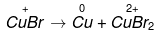It is an example of disproportionation reaction, as Cu undergoes both oxidation and reduction.

Q.60. The oxidation states of Cr in [Cr(H2O)6] Cl3 , [Cr(C6H6)2] and K2[Cr(CN)2 (O)2 (O2) NH] respectively are:    (2018)
(a) +3, +4, and +6
(b) +3, +2, and +4
(c) +3, 0, and +6
(d) +3, 0, and +4

Ans. c
The Oxidation state of Cr in
[Cr(H2O)6]CI3
x + 6(0) - 3 = 0
x = +3
[Cr(C6H6)2]
x + 2(0) = 0
x = 0
K2 [Cr (CN)2 (O)2 (O2) NH3]
+2 + x + 2 (-1) + 2 (-2) +1 (-2) + 0
= 6
∴ x = +6

Q.61. A sample of NaCIO3 is converted by heat to NaCl with a loss of 0.16 g of oxygen. The residue is dissolved in water and precipitated as AgCl. The mass of AgCI (in g) obtained will be: (Given: Molar mass of AgCl= 143.5 g mol -1)    (2018)
(a) 0.35
(b) 0.48
(c) 0.54
(d) 0.41

Ans. b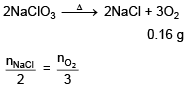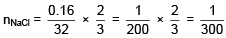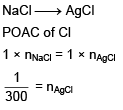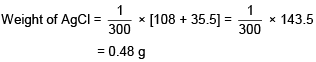Q.62. An unknown chlorohydrocarbon has 3.55 % of chlorine. If each molecule of the hydrocarbon has one chlorine atom only ; chlorine atoms present in 1 g of chlorohydrocarbon are:    (2018)
(Atomic wt. of Cl = 35.5 u ; Avogadro constant = 6.023 × 1023 mol–1)
(a) 6.023 × 109
(b) 6.023 × 1023
(c) 6.023 × 1021
(d) 6.023 × 1020

Ans. d
CxHyCl
% Cl = 3.55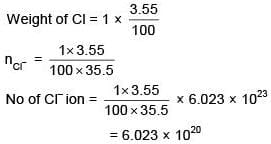Q.63. The most abundant elements by mass in the body of a healthy human adult are:
Oxygen (61.4%); Carbon (22.9%); Hydrogen (10.0%) and Nitrogen (2.6%).
The weight which a 75 kg person would gain if all 1H atoms are replaced by 2H atoms is    (2017)
(a) 15 kg
(b) 37.5 kg
(c) 7.5 kg
(d) 10 kg

Ans. c
Mass of hydrogen = 10/100 x 75 = 7.5 kg
Replacing 1H by 2H would replace 7.5 kg with 15 kg
∴ Net gain = 7.5 kg

Q.64. 1 gram of a carbonate (M2CO3) on treatment with excess HCl produces 0.01186 mole of CO2. The molar mass of M2CO3 in g mol-1 is    (2017)
(a) 1186
(b) 84.3
(c) 118.6
(d) 11.86

Ans. b
M2CO3 + 2HCl → 2MCl + H2O + CO2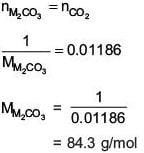Q.65. Excess of NaOH(aq) was added to 100 mL of FeCI3(aq) resulting into 2.14 g of Fe(OH)3. The molarity of FeCI3(aq) is:
(Given molar mass of Fe = 56 g mol-1 and molar mass of CI = 35.5 g mol-1)    (2017)
(a) 0.3 M
(b) 0.2 M
(c) 0.6 M
(d) 1.8 M

Ans. (b)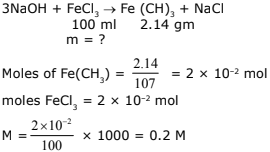Q.66. What quantity (in mL) of a 45% acid solution of a mono-protic strong acid must be mixed with a 20% solution of the same acid to produce 800 mL of a 29.875% acid solution?    (2017)
(a) 316
(b) 320
(c) 325
(d) 330

Ans. a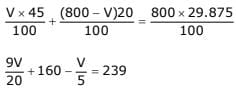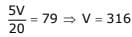Q.67. At 300 K and 1 atm, 15 mL of a gaseous hydrocarbon requires 375 mL air containing 20% O2 by volume for complete combustion. After combustion the gases occupy 330 mL. Assuming that the water formed is in liquid form and the volumes were measured at the same temperature and pressure, the formula of the hydrocarbon is:    (2016)
(a) C3H6
(b) C3H8
(c) C4H8
(d) C4H10

Ans. b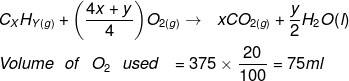From the reaction of combustion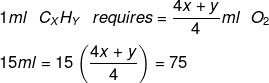So, 4x + y = 20
x = 3
y = 8
C3 H8

Q.68. 5L of an alkane requires 25 L of oxygen for its complete combustion. If all volumes are measured at constant temperature and pressure, the alkane is:    (2016)
(a) Ethane
(b) Propane
(c) Butane
(d) Isobutane

Ans. b
Combustion of Hydrocarbon: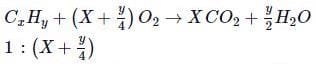∴ x = y/4 = 5 which is satisfied by C3 H8 (Propane)

Q.69. The amount of arsenic pentasulphide that can be obtained when 35.5 g arsenic acid is treated with excess H2S in the presence of conc. HCl (assuming 100% conversion)    (2016)
(a) 0.25 mol
(b) 0.125 mol
(c) 0.333 mol
(d) 0.50 mol

Ans. b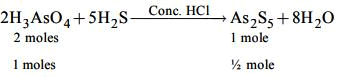∴ number of moles of H3AsO4
= 35.5/142 = 0.25
∴ number of moles of As2 S5
= 0.25/2 = 0.125 mole.

Q.70. The volume of 0.1 N dibasic acid sufficient to neutralize 1 g of a base that furnishes 0.04 mole of OH in aqueous solution is:    (2016)
(a) 400 mL
(b) 600 mL
(c) 200 mL
(d) 80 mL

Ans. c
As we know that in a basic acid there is one ionizable hydrogen. While in the dibasic acid there are two ionizable hydrogen. In the given data there are 0.04 moles of OH- ion in aqueous solution. The volume of the solution is found below:
V × 0.1 × 2 = 0.04
V = 200 ml

The document JEE Main Previous year questions (2016-22): Some Basic Concepts of Chemistry Notes | Study Chemistry for JEE - JEE is a part of the JEE Course Chemistry for JEE.
All you need of JEE at this link: JEE

## Chemistry for JEE

298 videos|517 docs|369 tests
 Use Code STAYHOME200 and get INR 200 additional OFF

## Chemistry for JEE

298 videos|517 docs|369 tests

Track your progress, build streaks, highlight & save important lessons and more!

,

,

,

,

,

,

,

,

,

,

,

,

,

,

,

,

,

,

,

,

,

;